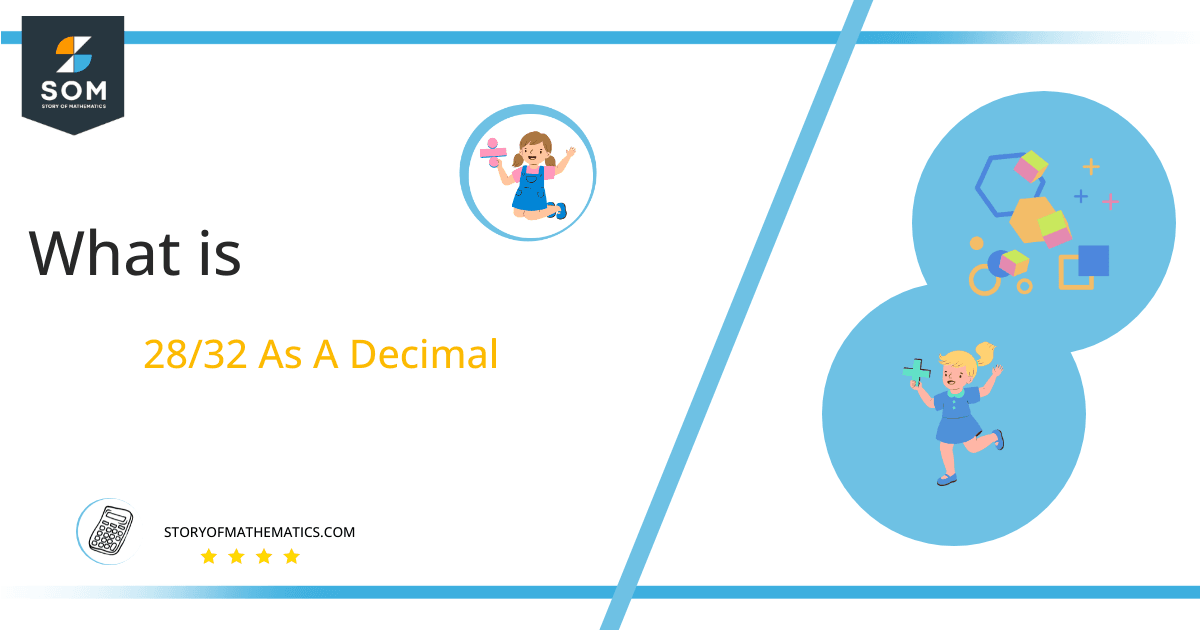# What Is 28/32 as a Decimal + Solution With Free Steps

The fraction 28/32 as a decimal is equal to 0.875.

A Decimal Number is converted into a Fraction by placing “1” and “0’s” in the denominator. e.g. 0.67 is a decimal number and 67/100 is the fraction expression. Here decimal number becomes the numerator and in the denominator, we used 1 in place of the decimal point and write the number of zeros as the number of digits comes after the decimal point. Write 1 with two 0’s in the denominator because 2 digits are used after the decimal point.

Here, we are more interested in the division types that result in a Decimal value, as this can be expressed as a Fraction. We see fractions as a way of showing two numbers having the operation of Division between them that result in a value that lies between two Integers.Now, we introduce the method used to solve said fraction to decimal conversion, called Long Division, which we will discuss in detail moving forward. So, let’s go through the Solution of fraction 28/32.

## Solution

First, we convert the fraction components, i.e., the numerator and the denominator, and transform them into the division constituents, i.e., the Dividend and the Divisor, respectively.

This can be done as follows:

Dividend = 28

Divisor = 32

Now, we introduce the most important quantity in our division process: the Quotient. The value represents the Solution to our division and can be expressed as having the following relationship with the Division constituents:

Quotient = Dividend $\div$ Divisor = 28 $\div$ 32

This is when we go through the Long Division solution to our problem. The following figure shows the long division:Figure 1

## 28/32 Long Division Method

We start solving a problem using the Long Division Method by first taking apart the division’s components and comparing them. As we have 28 and 32, we can see how 28 is Smaller than 32, and to solve this division, we require that 28 be Bigger than 32.

This is done by multiplying the dividend by 10 and checking whether it is bigger than the divisor or not. If so, we calculate the Multiple of the divisor closest to the dividend and subtract it from the Dividend. This produces the Remainder, which we then use as the dividend later.

Now, we begin solving for our dividend 28, which after getting multiplied by 10 becomes 280.

We take this 280 and divide it by 32; this can be done as follows:

280 $\div$ 32 $\approx$ 8

Where:

32 x 8 = 256

This will lead to the generation of a Remainder equal to 280 – 256 = 24. Now this means we have to repeat the process by Converting the 24 into 240 and solving for that:

240 $\div$ 32 $\approx$ 7

Where:

32 x 7 = 224

This, therefore, produces another Remainder which is equal to 240 – 224 = 16. Now we must solve this problem to Third Decimal Place for accuracy, so we repeat the process with dividend 160.

160 $\div$ 32 = 5

Where:

32 x 5 = 160

Finally, we have a Quotient generated after combining the three pieces of it as 0.875=z, with a Remainder equal to 0.Images/mathematical drawings are created with GeoGebra.